# Discounting

Finding the present value of a sum of money or a series of cash flows received in the future

Discounting is a crucial idea in finance which includes finding the present value of a sum of money or a series of cash flows received in the future. It revolves around a recurring financial motif called the time value of money.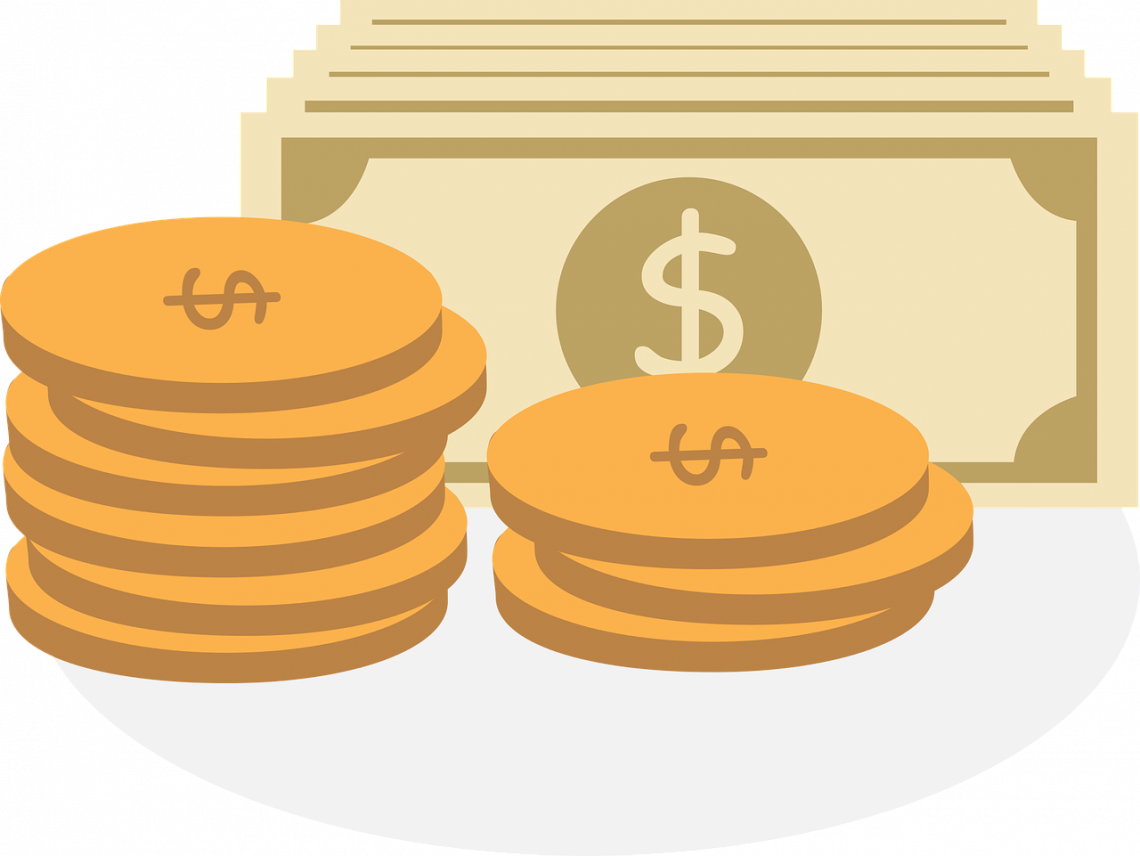This notion in finance means that a dollar in your pocket today is worth more than a dollar in your hands in the future. That is because if you can earn interest on that dollar that you have today while you wait for it to grow.

One dollar we have today invested in the right place will grow to become more than a dollar in the future. That is why it is always preferable to have a dollar right now than to have a dollar in the future. Therefore, consumers and investors should always consider their decisions regarding spending or investing their money.

It is also coherent with the idea of the present value of an asset, a sum of money, or a stream of future cash flows. When we discount any of these, we are essentially finding the present value of these assets at an earlier date, particularly the current date.

Present value is a crucial concept to understand for every investor and manager. For example, someone may want to know how much money they should invest now to have a certain amount of money in 10 years.

That question is answered by discounting the amount of money the person has in mind by 10 years. Naturally, the present value of that sum of money will be much less than the amount of money needed at the end.

That is because a dollar today is more valuable than a dollar after 10 years. Of course, the investor must have a specific interest rate in mind - the discount rate - which will be used to discount the sum of money to its present value.

## Understanding Discounting

This means transforming the future value of a sum of money or a series of cash flows into its present value: how much it would be worth today. Discounting is the opposite of compounding, which means transforming the present value into its future value.It is essential in determining the price of different assets. The price of an asset that provides cash flows in the future is the discount of those cash flows. Discounting deals with price problems depending on a company's potential future financial success.

Take stocks, for example. Because the value or price of an asset is the present value of its future cash flows, we can theoretically find the price of a stock. The future cash flows a stock can have are called dividend payments.

So what is the current value of the stock? To find the price of a share, all we have to do is discount its future cash flows - that is, the dividends - to the current date. This can provide insights into how to value a stock based on the announced dividends that it will pay.

Stocks can pay different amounts of dividends or have equal dividend payments yearly. When a share pays equal dividends across several years, it is called an annuity payment. Alternatively, it can have a growing dividend at a certain percentage each year.

In addition, equal dividends can be paid throughout the corporation's life forever. This is called payments in perpetuity (coming from the word perpetual). Finally, there is a method to discount each payment using different formulas, all of which will be discussed later.

Another vital asset whose price or current value can be determined by discounting is bonds. Bonds have a face value, also called par or maturity value which describes the bond's value when the bond is first issued.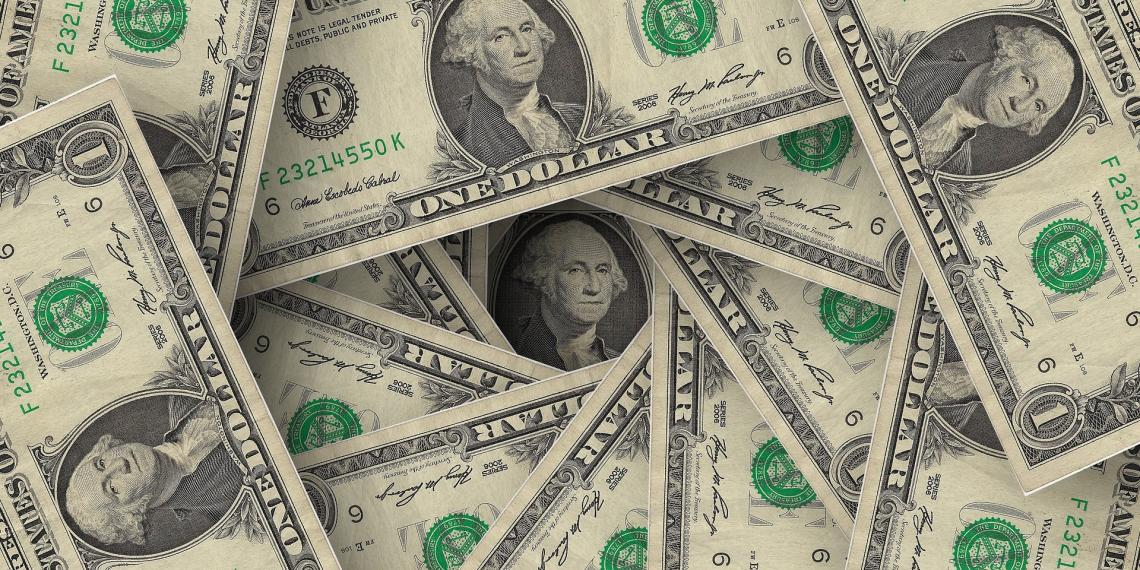It is also called maturity value because it totals the principal amount paid back to the holder at the end of maturity. Bonds also have interest payments, known as coupon payments.

To find the price at which the bonds are traded, we should discount the future coupon payments to find their present value. In addition, we should also discount the face value of \$1,000 to the current date and add it to the present value of the stream of coupon payments.

## Formulas for Discounting

There are multiple formulas to be able to discount future cash flows to the current date. All of these formulas almost have the same variables arranged differently: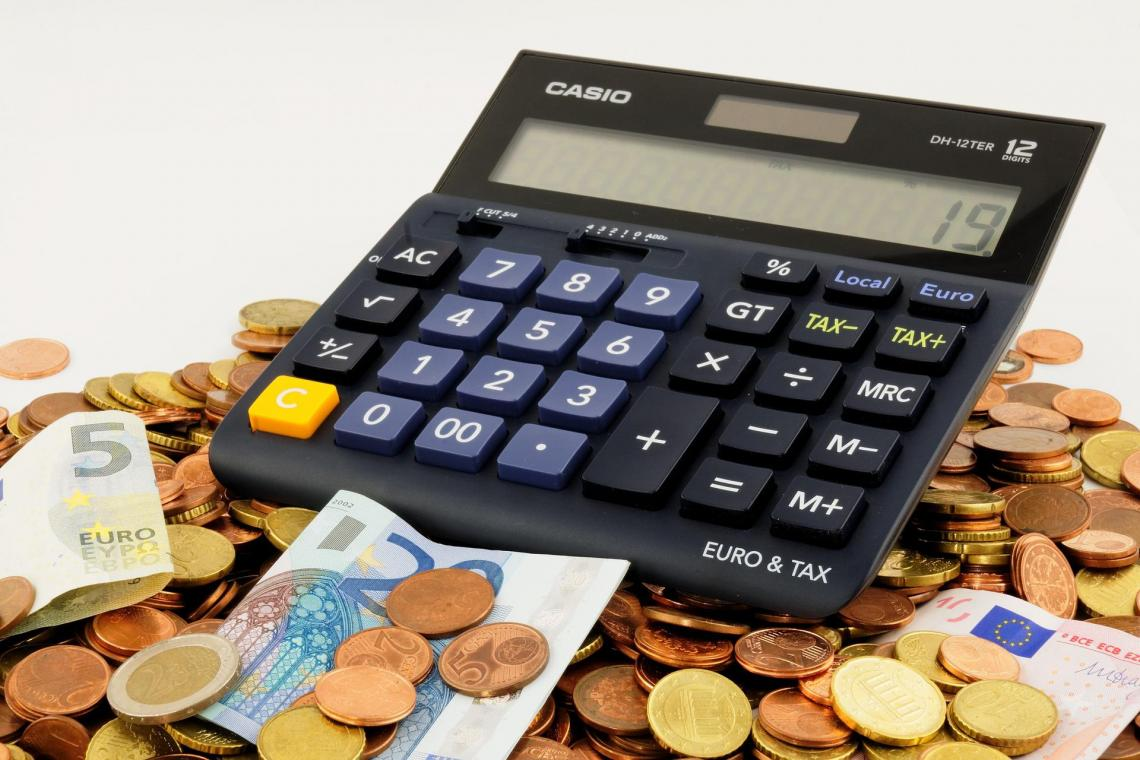• Present value (which can also be referred to as principal or balance),

• Future value (which can also be referred to as face value),

• Periodic payments, as in the case of annuities and perpetuities (which can also be referred to as coupons),

• Interest rate (which can also be referred to as discount rates),

• The number of periods.

The most fundamental formula when it comes to discounting is the present value of a lump of money to be received in one period into the future: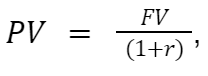• PV: present value, the current value of an amount of money or a series of cash flows, is discounted using a predetermined discount rate.

• FV: future value, which is the value of an asset or a sum of money at a future date, given that asset's present value.

• r: the discount rate, the rate at which future values are discounted to the current period.

If the sum of the money were to be received after more than one period, then the formula would undergo a minor addition:"n" is the number of periods after which a lump sum of money will be received. This formula is the one that most accurately represents the time value of money. This formula can also find the total present value of multiple future cash flows in different periods.The following formula is important in discounting an annuity payment. An annuity is characterized by same-sized payments equally spaced between two points in time. It is a stream of equal and periodic payments. The formula for the present value of an annuity is: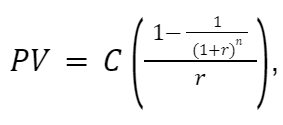Multiple variables need to be labeled in this formula:

• C represents the periodic cash inflows in which annuities are characterized.

• The variable "n" represents the number of periods, the same as the number of payments occurring throughout the annuity.

• r represents the discount rate

Knowing the three variables "C," "n," and "r" will suffice to calculate the present value of an annuity. Nevertheless, we can also find the values of any of those variables as long as we know the present value of the annuity.

One thing to keep in mind when calculating annuities is the timing of the periodic payments. In the formula, it is assumed that payments are being made or received at the end of each period. This usual case is known as an ordinary annuity.

If payments are made at the beginning of each period, this is known as an annuity due, and the calculations slightly differ to discount an annuity due. We just have to multiply the PV of an ordinary annuity by (1+r), and we will have the present value of an annuity due.The final formula important in discounting is being able to discount a perpetuity payment.

Perpetuity is similar to an annuity because the cash inflows are equal and periodically spaced. The difference is that perpetuities go on forever (coming from the word perpetual).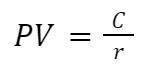This formula can be used in stock valuation. For example, some corporations promise their shareholders dividends in perpetuity, so this formula is suitable to estimate the current value of that stock. This is also known as the Dividend Discount Model or the Gordon Growth Model.

## Notes

1. All the equations above can be rearranged to solve for variables other than the present value.

2. The discount rate r is denominated for the relevant period. That is to say. When payments are made yearly, the interest is denominated as an annual interest rate. If payments were made monthly, the interest rate should be divided by 12, and the number of periods should be multiplied by 12.

3. Usually, it is impossible to determine the discount rate r by rearranging the formulas; it requires a financial calculator or spreadsheet software like Excel

## Understanding the Discount Rate

In short, the discount rate is the interest rate used to find the present value of future cash flows. It is a percentage applied in discounted cash flow analysis (DCF). It can also have other meanings, such as the rate the Fed charges to banks for short-term loans.However, the first meaning of the discount rate is the one of interest in our topic. It is the interest rate used to find the present value of the expected future cash flows. For example, discounting \$1,100 in one year to the current date using a discount rate of 10% yields a PV of \$1,000.

Every investor and financial manager has to decide upon a reasonable and accurate discount rate to find the present value of investments. Once the discount rate is decided upon, estimating future cash flows will enable them to calculate the PV of such projects.

An appropriate discount rate is crucial in DCF since an investor can make erroneous decisions after calculating the present value of an investment. After all, the PV of a project indicates how much value it creates and how profitable it can be.

For assets that don't carry risks above normal levels, the most appropriate rate that can be used is the risk-free rate. This rate represents the return of an investment or asset with zero risk. It is typically equal to the interest rate on 3-month US treasury bills.

On the corporate level, the most commonly used discount rate is the weighted average cost of capital (WACC). This is because companies usually invest in assets and projects with more risk than safer ones, like treasury bills.

WACC is inherently a percentage that shows us the company's cost to acquire new sources of finance, mainly debt and equity. That is why it is a weighted average between using debt and giving out equity to finance projects.

## Practical examples of discounting

The two main real-life examples that will be discussed are finding the value of a stock and finding the value of a bond. For the stock, we will use the perpetuity formula, and for the bond, we will use the annuity formula.1. Suppose a preferred stock pays \$1 every quarter to its shareholders. At a discount rate of 10%, at what price should the stock sell today? This situation represents perpetuity since equal payments are being made indefinitely. Recall the formula of the PV of perpetuity.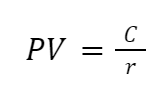C is the number of periodic payments, and r represents the discount rate. Since the payments are made quarterly, we must change the denomination of the interest rate from a yearly to a quarterly rate. This is done by dividing the 10% by 4 to get 2.5%.

Therefore, the stock should sell for: PV = \$1 / 0.025 = \$40.

2. What is the value of a bond with a face value of \$1,000 that pays a semiannual coupon with a coupon rate of 14%? The bond matures in 7 years, and the market's yield to maturity (the discount rate) is 16%.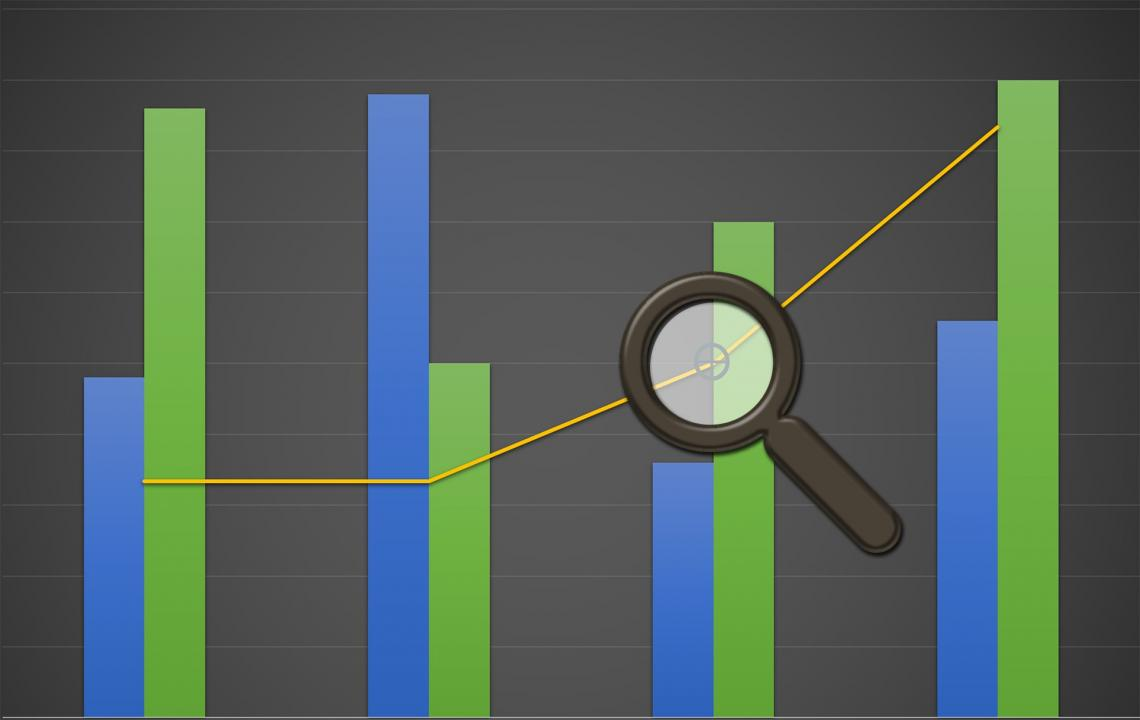Most bonds have two types of payments. The first is the stream of coupon payments (annuity). With a coupon rate of 14% on the \$1,000 face value, the bond pays \$140 coupons per year. Since the payments are semiannual, the bond pays \$70 every six months.

The second type of payment is future repayment. It represents a lump-sum return of capital of \$1,000 after seven years when the bond matures. Therefore, finding the value of the bond requires combining the annuity and the lump-sum present value formulas. Recall the formulas: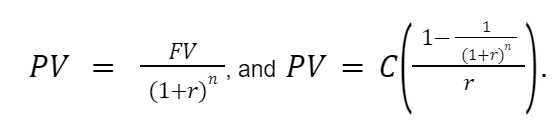Remember that we should transform the 16% discount rate into a semiannual rate by dividing it by two and multiplying the number of periods (7) by 2. First, we calculate the present value of the \$1,000 on the current date, which is equal to: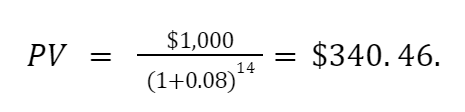Then, we calculate the present value of the stream of coupon payments in the form of an annuity: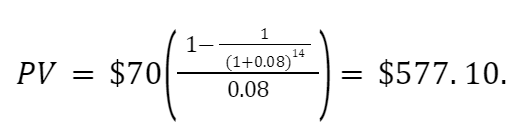Therefore, the bond's current price is the sum of the two calculated present values. Therefore, the bond should currently sell for: \$340.46 + \$577.10 = \$917.56.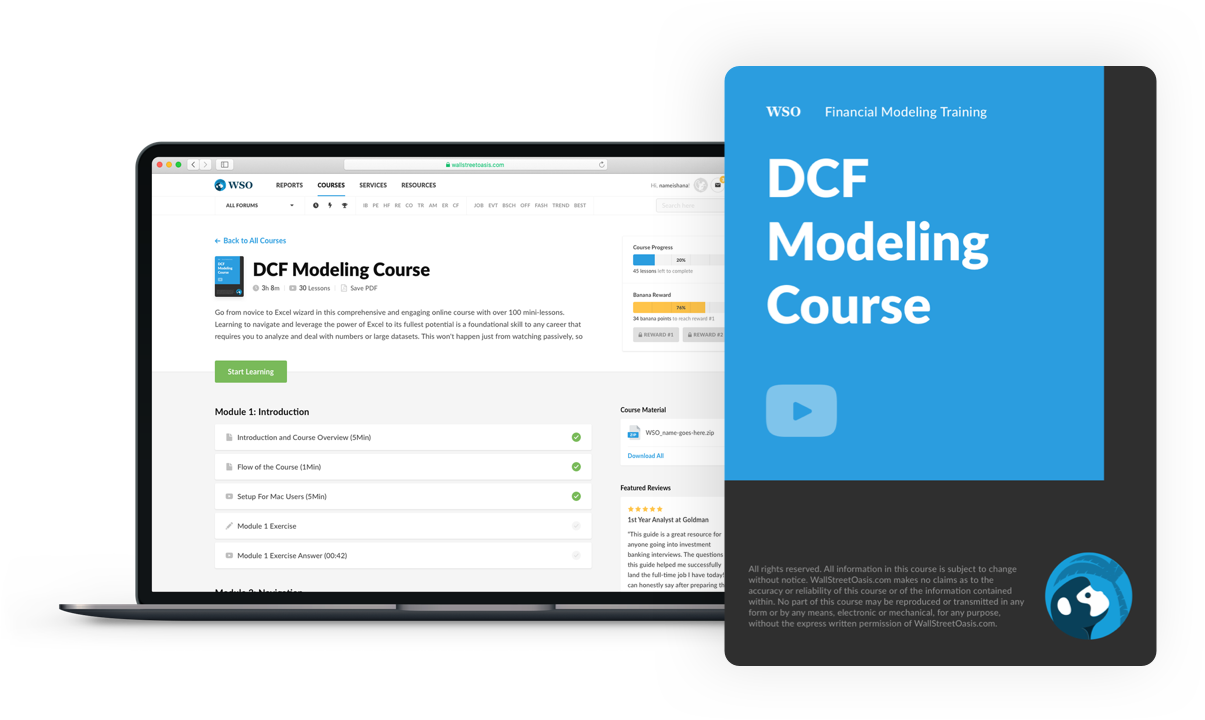### Everything You Need To Master DCF Modeling

To Help You Thrive in the Most Prestigious Jobs on Wall Street.

Researched and Authored by Vatche Tchelderian | LinkedIn